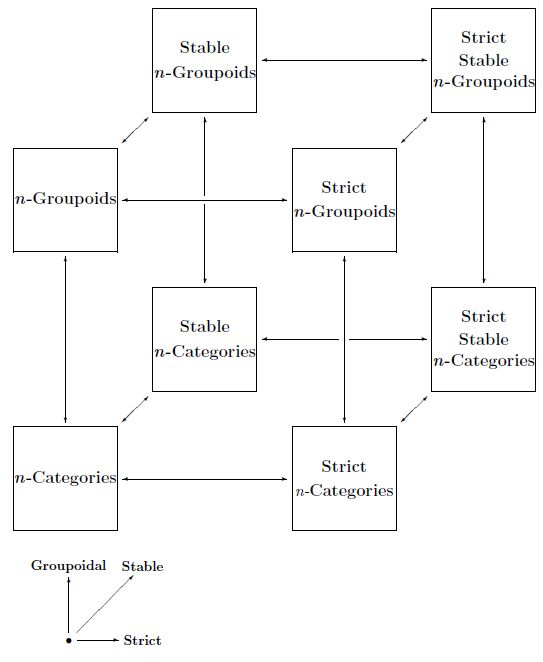# nLab cosmic cube

Contents

### Context

#### Higher category theory

higher category theory

# Contents

## Idea

The Cosmic Cube of higher category theory is the name for a diagram whose vertices correspond to special types of n-categories. The cube looks like this:We may take $n = \infty$ here as well, and we may also consider a version for (n,r)-categories. The three axes correspond to:

• making $n$-categories ‘groupoidal’ — that is, making morphisms invertible, thus passing from general $n$-categories to n-groupoids;

• making $n$-categories strict, thus passing from general $n$-categories to strict $n$-categories;

• making $n$-categories symmetric monoidal or ‘stable’, thus passing from general $n$-categories to symmetric monoidal $n$-categories.

### In terms of homotopy theory

Each vertex of the cube can also be understood as corresponding to a version of homotopy theory:

$\infty$-groupoids yield ordinary homotopy theory, symmetric monoidal and groupal $\infty$-groupoids correspond to stable homotopy theory, strictly abelian strict $\infty$-groupoids correspond to homological algebra. $\infty$-Categories that are not $\infty$-groupoids correspond to directed homotopy theory.

## Vertices of the cube

Here we list the 8 vertices of the cube in the case of $\infty$-categories.

(…)

## Edges of the cube

### Strict $\infty$-groupoids in all $\infty$-groupoids

A strict ∞-groupoid is modeled by a crossed complex. Under ∞-nerve it embeds into all ∞-groupoids, modeled as Kan complexes.

$\array{ CrsCplx &\stackrel{N^\Delta}{\hookrightarrow}& KanCplx \\ \downarrow && \downarrow \\ Str \infty Grpd &\hookrightarrow& \infty Grpd } \,.$

### Strictly stable strict $\infty$-groupoids in strict $\infty$-groupoids

A strictly stable strict ∞-groupoid is modeled by a bounded-below chain complex of abelian groups. Under the embedding $\Theta$ of complexes into crossed complexes it embeds into strict ∞-groupoids.

$\array{ ChnCplx &\stackrel{\Theta}{\hookrightarrow}& CrsCplx \\ \downarrow && \downarrow \\ StrAb Str \infty Grpd &\hookrightarrow& Str \infty Grpd } \,.$

For the definition of $\Theta$ see Nonabelian Algebraic Topology , section Crossed complexes from chain complexes.

### Strictly stable strict $\infty$-groupoids in all $\infty$-groupoids

Combining the above inclusions

$\array{ ChainCplx &\stackrel{\Theta}{\hookrightarrow}& CrossedCplx &\stackrel{N^\Delta}{\hookrightarrow}& KanCplx \\ \downarrow^{\mathrlap{\simeq}} && \downarrow^{\mathrlap{\simeq}} && \downarrow^{\mathrlap{\simeq}} \\ StrAb Str\infty Grpd &\hookrightarrow& Str \infty Grpd &\hookrightarrow& \infty Grpd }$

yields in total the map $ChnCplx \to sAb$ from chain complexes to simplicial abelian groups (followed by the forgetful $sAb \to KanCpx$) of the Dold-Kan correspondence.

### Strictly stable strict $\infty$-groupoids in strictly stable $\infty$-groupoids

A strictly stable strict ∞-groupoid is modeled by a bounded-below chain complex of abelian groups. Under ∞-nerve it embeds into all (connective) spectras, modeled as spectrum objects in Kan complexes.

$\array{ ChnCplx^+ &\stackrel{\Sigma^\infty \N^\Delta \Theta}{\hookrightarrow}& Sp(KanCplx) \\ \downarrow && \downarrow \\ StrAb Str \infty Grpd &\hookrightarrow& Sp(\infty Grpd) } \,.$

### Strictly stable $\infty$-groupoids in all $\infty$-groupoids

A strictly stable ∞-groupoid is modeled by a connective spectrum. The forgetful functor to ∞-groupoids is also called $\Omega^\infty$ or the “zeroth-space functor.”